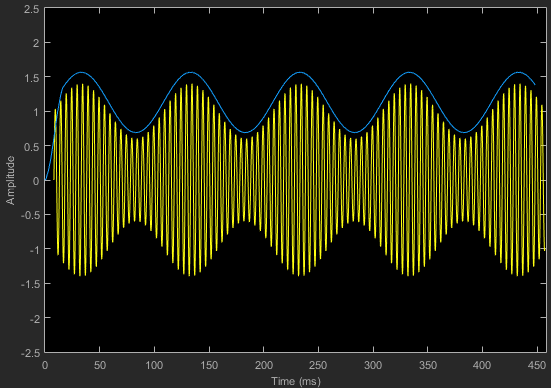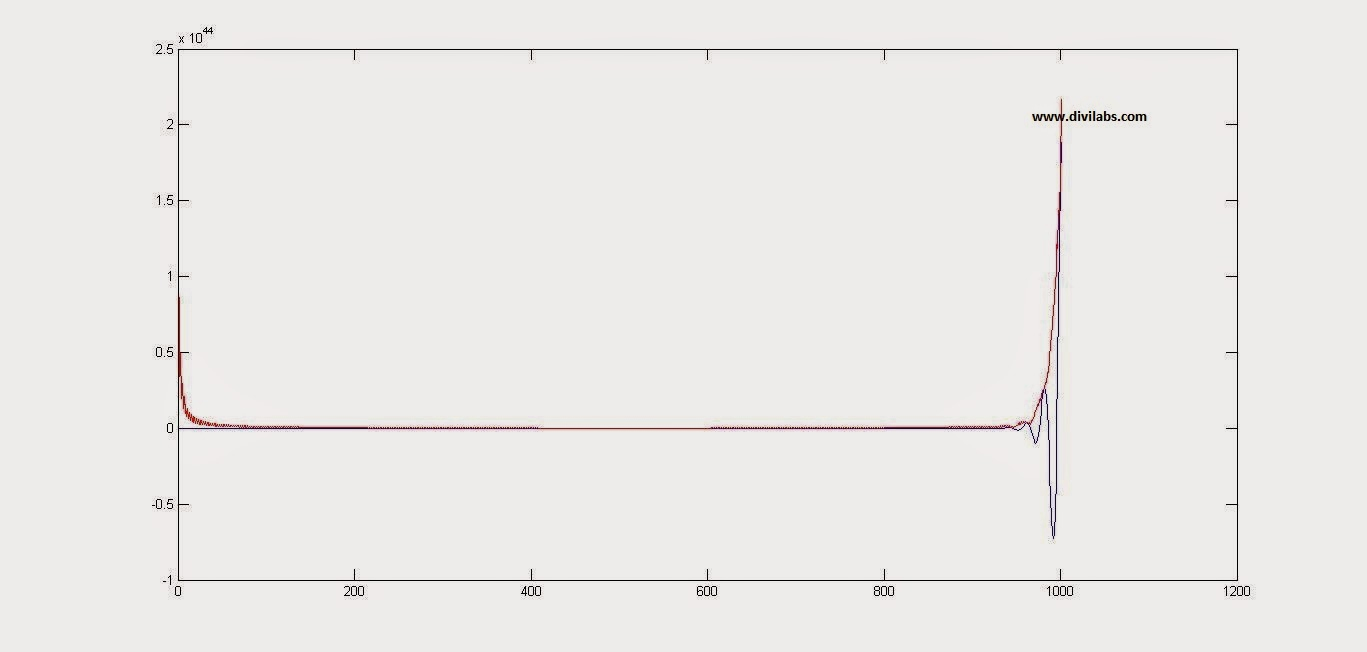# Envelope Detection Using MATLAB for Any time Domain Signal (Thorugh Hilbert Transform)Envelope Detection Using MATLAB for Any time Domain Signal
The above diagram/plot is of an Amplitude Modulated wave, & we have just found its envelope. This was the result of one of the MATHWORK'S example in simulink to demodulate the AM signal.

Electronically envelope detector is a very simple circuit, consisting of a diode, resistor & capacitor. From ages it has been used to De-modulate the amplitude modulated signals, on the receiver side. The output of the circuit is approximately a voltage-shifted version of the original baseband signal. A simple filter (like a capacitor) can be used to filter out the DC component. In order to get the desired amplitude variation.

That was a little about the circuit implementation of Envelope Detector, but how through a MATLAB code we are going to implement the envelope detection?Envelope Detection Using MATLAB
The answer lies hidden in the Hilbert Transform. It been a very common and efficient technique for envelope detection. The Hilbert transform is typically implemented as an FIR filter so the original signal must be delayed to match the group delay of the Hilbert transform. This process can be followed by absolute and then peak hold functions.

Another option for detecting the envelope is to use the square root of the energies of the original and the Hilbert transformed signals, as follows :
Envelope = sqrt(Hilbert^2 + signal^2)
In general, this will give similar results to the absolute value technique but can be more run-time efficient.

So, in order to do it, in MATLAB just the simplest way to add this line after the signal of which the envelope has to be calculated.
envelope = abs(hilbert(Signal));
We will show with the help of example,

Demodulating the AM Signal, using Envelope Detection through MATLAB.
clc;
clear all;
close all;

Ac=2; %carrier amplitude
fc=0.5; %carrier frequency
Am=.5; %message signal amplitude
fm=.05; %message signal frequency
Fs=100; %sampling rate/frequency

ka=1; %modulation coefficient

t=[0:0.1:100]; %defining the time range & disseminating it into samples
ct=Ac*cos(2*pi*fc*t); %defining the carrier signal wave
mt=Am*cos(2*pi*fm*t); %defining the message signal
AM=ct.*(1+ka*mt); %Amplitude Modulated wave, according to the standard definition

plot(AM, 'b');
hold on;
envelope = abs(hilbert(AM));
% hilbert transform to calculate the envelope of the signal
plot(envelope, 'r');
hold off

The result is:Envelope Detection Using MATLAB for Amplitude Modulated Wave (AM Demodulation)
Another similar result we have obtained by applying it to a product of exponential & cosine signal. The code & result as follows:
clc;
clear all;
close all;

Ac=2; %carrier amplitude
fc=0.5; %carrier frequency
Am=3.5; %message signal amplitude
fm=.05; %message signal frequency
Fs=100; %sampling rate/frequency

ka=1; %modulation coefficient

t=[0:0.1:100]; %defining the time range & disseminating it into samples
ct=Ac*cos(2*pi*fc*t); %defining the carrier signal wave
mt=Am*exp(t); %defining the message signal
AM=ct.*mt; %Amplitude Modulated wave, according to the standard definition

plot(AM, 'b');
hold on;
envelope = abs(hilbert(AM)); % hilbert transform to calculate the envelope of the signal
plot(envelope, 'r');
hold off
The result is:Envelope Detection Using MATLAB for Any time Domain Signal (Thorugh Hilbert Transform)